# The Weird World of Infinity in JavaScriptThe world of endlessness in JavaScript — explore the Infinity keyword .`Infinity`is a numeric value in JavaScript and corresponds to the mathematical representation of infinity. Technically, `Infinity` is classified as a property of the `window`object, similar to how variables in the global scope become properties of `window`

## What is Infinity in JavaScript?

`Infinity`is a numeric value in JavaScript and corresponds to the mathematical representation of infinity. Technically, `Infinity` is classified as a property of the `window`object, similar to how variables in the global scope become properties of `window`. The value of `Infinity` (positive infinity) is greater than any other number. Likewise, the value of `-Infinity` (negative infinity) is smaller than any other number.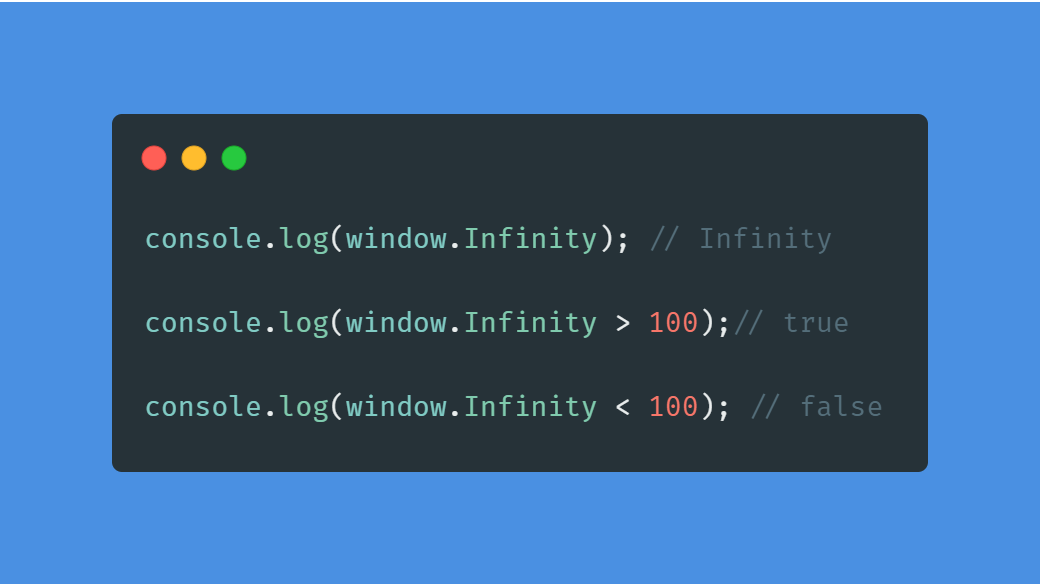Despite being a numerical value, `Infinity` cannot be iterated upon. For example, you would not be able to run it as a `for... of` loop. You will be returned with an `Uncaught TypeError: Infinity is not iterable` error.

``````// This won't work!
// Uncaught TypeError: Infinity is not iterable
for (let i of Infinity) {
console.log(i);
}
``````

But you can use the `Infinity` object as a ceiling or floor in your `for` loops. Although this is not advisable as it will probably crash your PC.

``````// Don't do this!
for (let i=0; i<Infinity; i++){
// Infinite loop
}
// Don't do this!
for (let i=0; i>-Infinity; i--){
// Infinite loop
}
``````

## When does Infinity occur?

If you console values and see for yourself, the console would display Infinity just as your number hits 309 digits regardless of the digit value(i.e: even 309 ones would be Infinity).

To be even more precise, according to the spec, `**Infinity**` represents all values greater than `**1.7976931348623157e+308**` .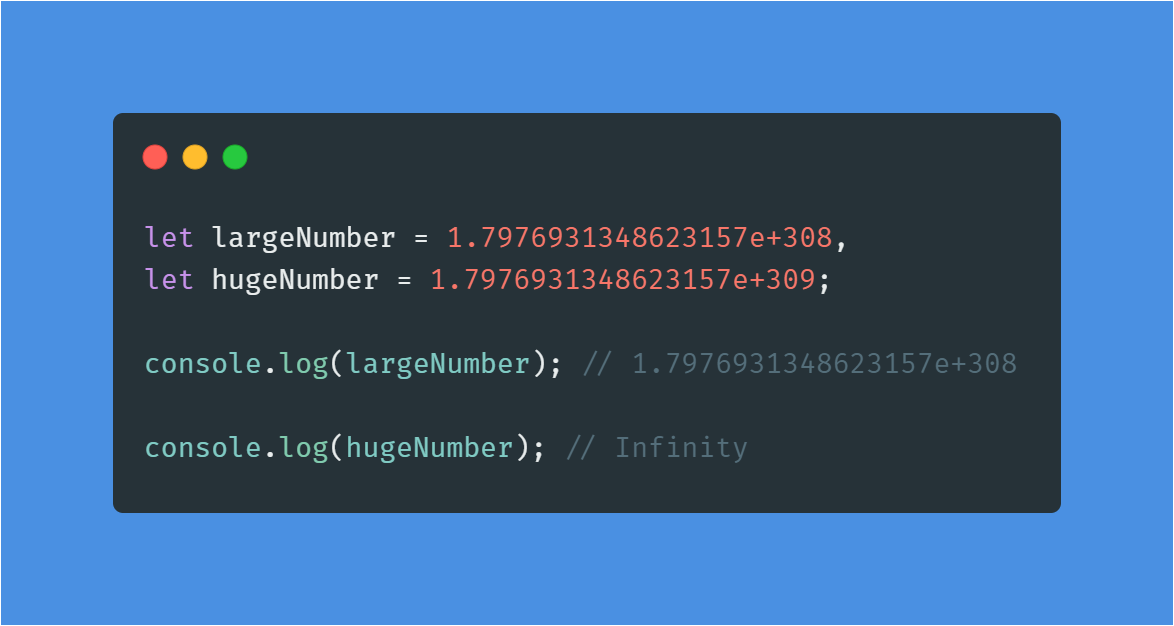Another instance where you will get Infinity as output is when you divide a number by zero.

``````console.log(90 / 0); // Infinity
console.log(-90 / 0); // -Infinity
``````

This is different from what you see on a calculator as you would receive a “cannot divide by zero” or “undefined” type of error.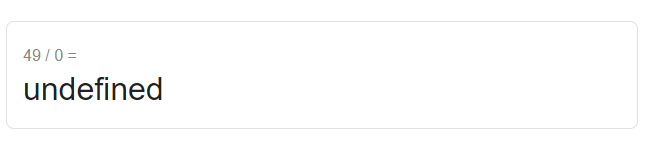Another way of receiving `Infinity` is by accessing them directly from the `Number` object in JavaScript.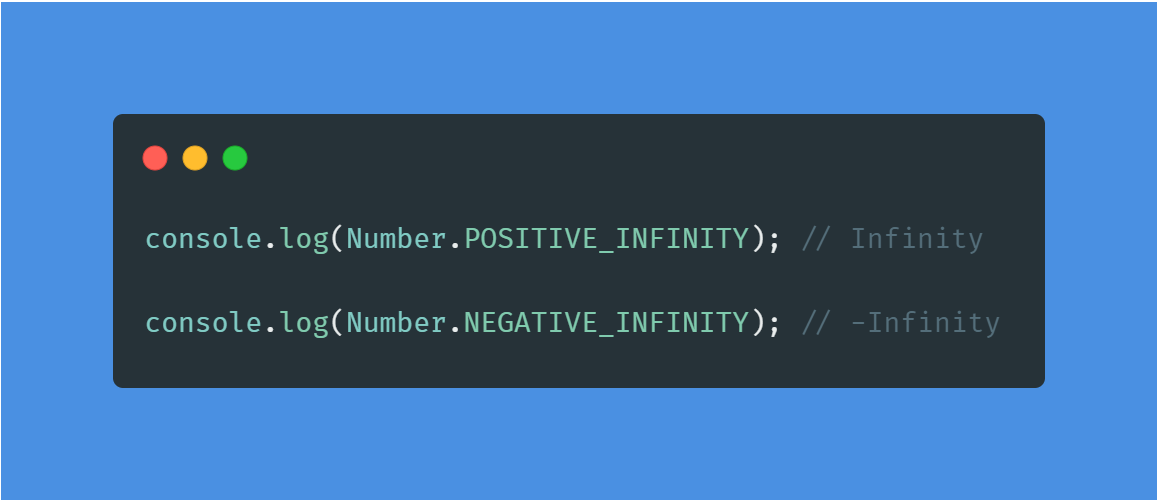## How to check for Infinity?

You can use your own function like below. This method checks for both Positive and Negative `Infinity`.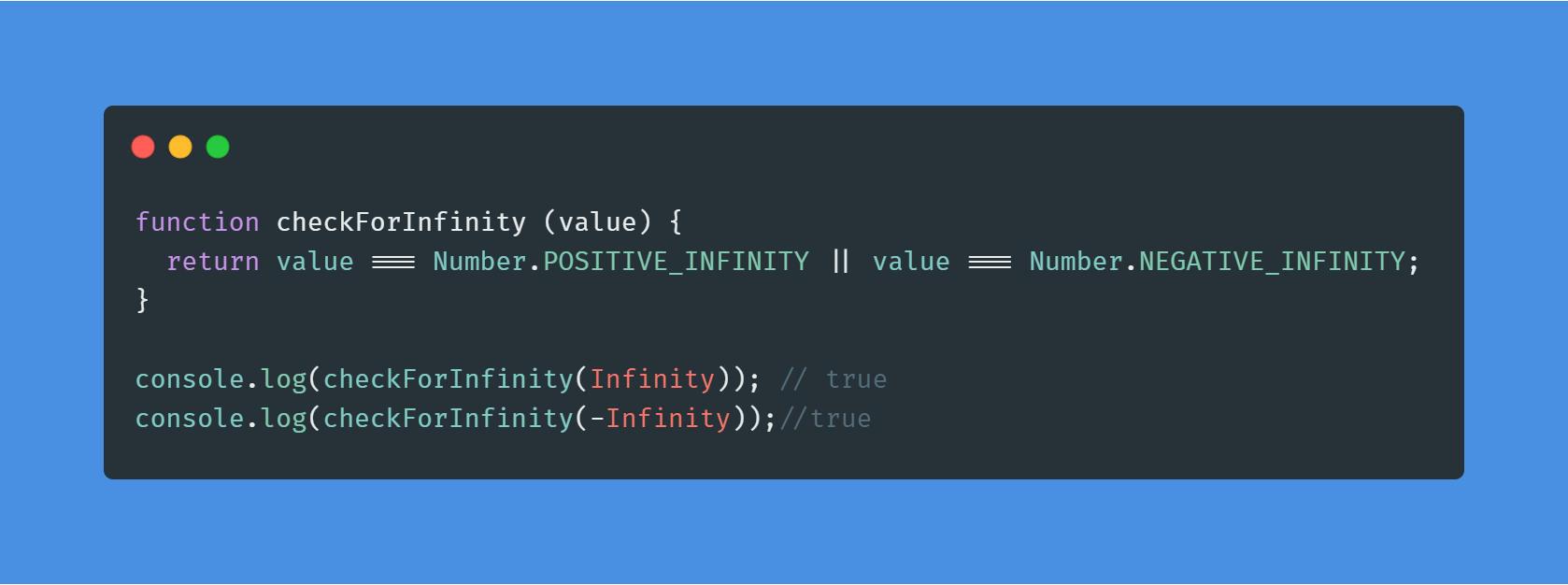If you want to go for a builtin JS method, you can go with the `isFinite()` method. The `isFinite()` function determines whether a number is a finite, legal number. This function returns false if the value is +Infinity, -Infinity, or NaN (Not-a-Number), otherwise it returns true.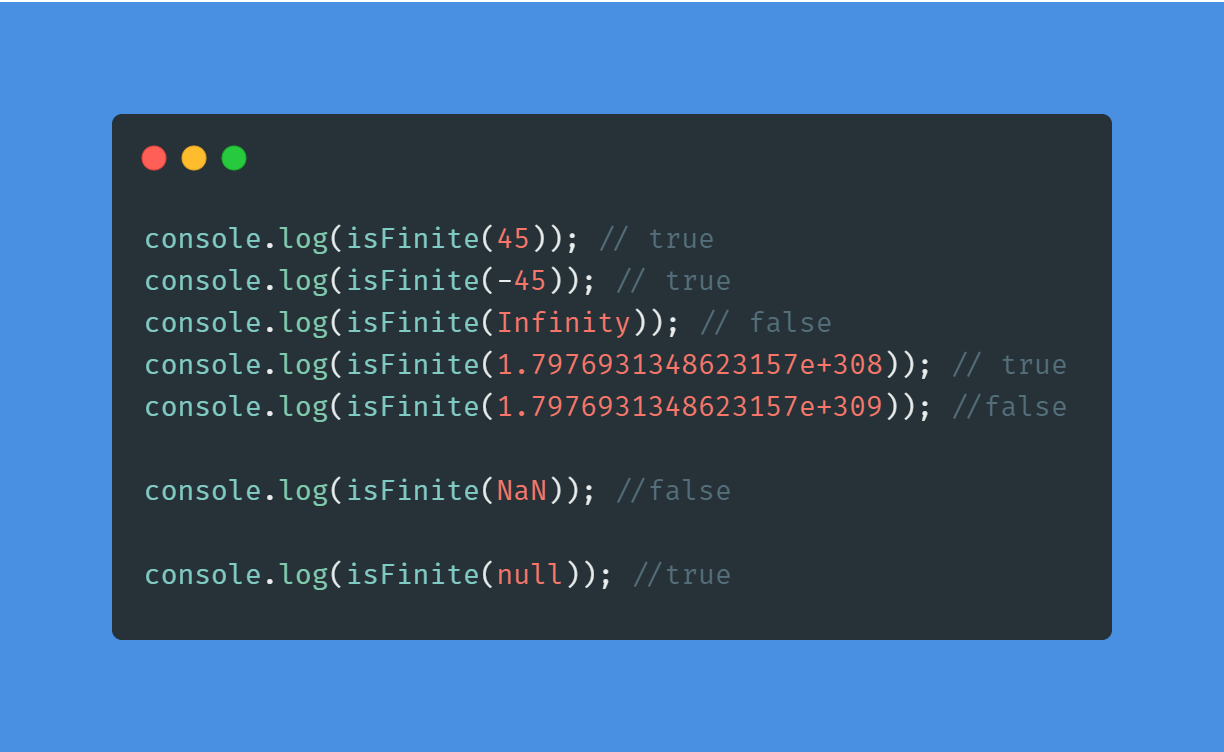NOTE
The
`**isFinite()**` method returns true for `**null**` . You must be cautious about this. It even coerces number string into number and returns true. But a non-number string will return false.

``````console.log(isFinite(null)); //true
console.log(isFinite('45')); // true
console.log(isFinite('-75')); // true
console.log(isFinite('hello')); //false
``````

## Where can you use Infinity?

In his new book JavaScript for impatient programmers, Axel Rauschmayer has an interesting use case for `Infinity` as a default value. Since `Infinity` is larger than all numbers, it can be useful in a function that checks for the minimum number in an array:

``````function findMinimum(numbers) {
let min = Infinity;
for (const n of numbers) {
if (n < min) min = n;
}
return min;
}
console.log(findMinimum([20, 6, 90])); // 6
``````

This works nicely because `Infinity` is greater than all numbers so unless all the numbers in the array cross the `Infinity` threshold, the result won’t have any problems.

## Things to be noted

When working with JSON data, make sure that you are aware of the data that is inside the object before you stringify the JSON object (`JSON.stringify()`). Take a look at this example.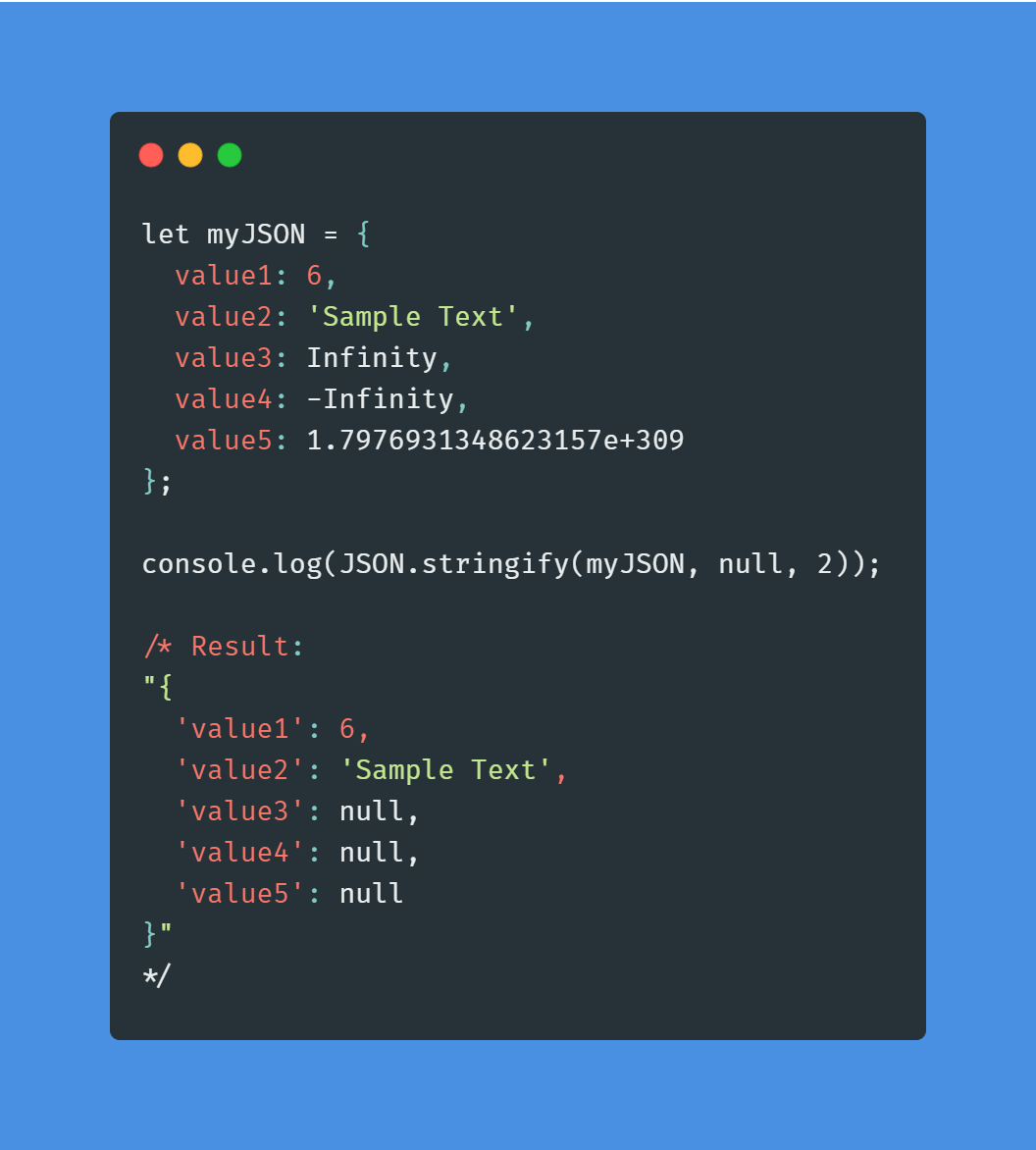As you can see, the `Infinity` values are converted to null when stringifying the JSON object. This would also happen if one of the values was initially `NaN`.

If I have missed anything, please do let me know in the comments.

Happy Coding!!